# Selina Solutions Concise Maths Class 10 Chapter 16 Loci (Locus and its Constructions) Exercise 16(A)

The Selina Solutions for Class 10 Maths is a one-stop solution to all doubts and clarifications in solving problems of this Chapter and others. All the solutions are as per the latest ICSE examination patterns. The solutions of this exercise are available in the Selina Solutions Concise Maths Class 10 Chapter 16 Loci (Locus and its Constructions) Exercise 16(A) PDF, provided in the links given below.

## Selina Solutions Concise Maths Class 10 Chapter 16 Loci (Locus and its Constructions) Exercise 16(A) Download PDF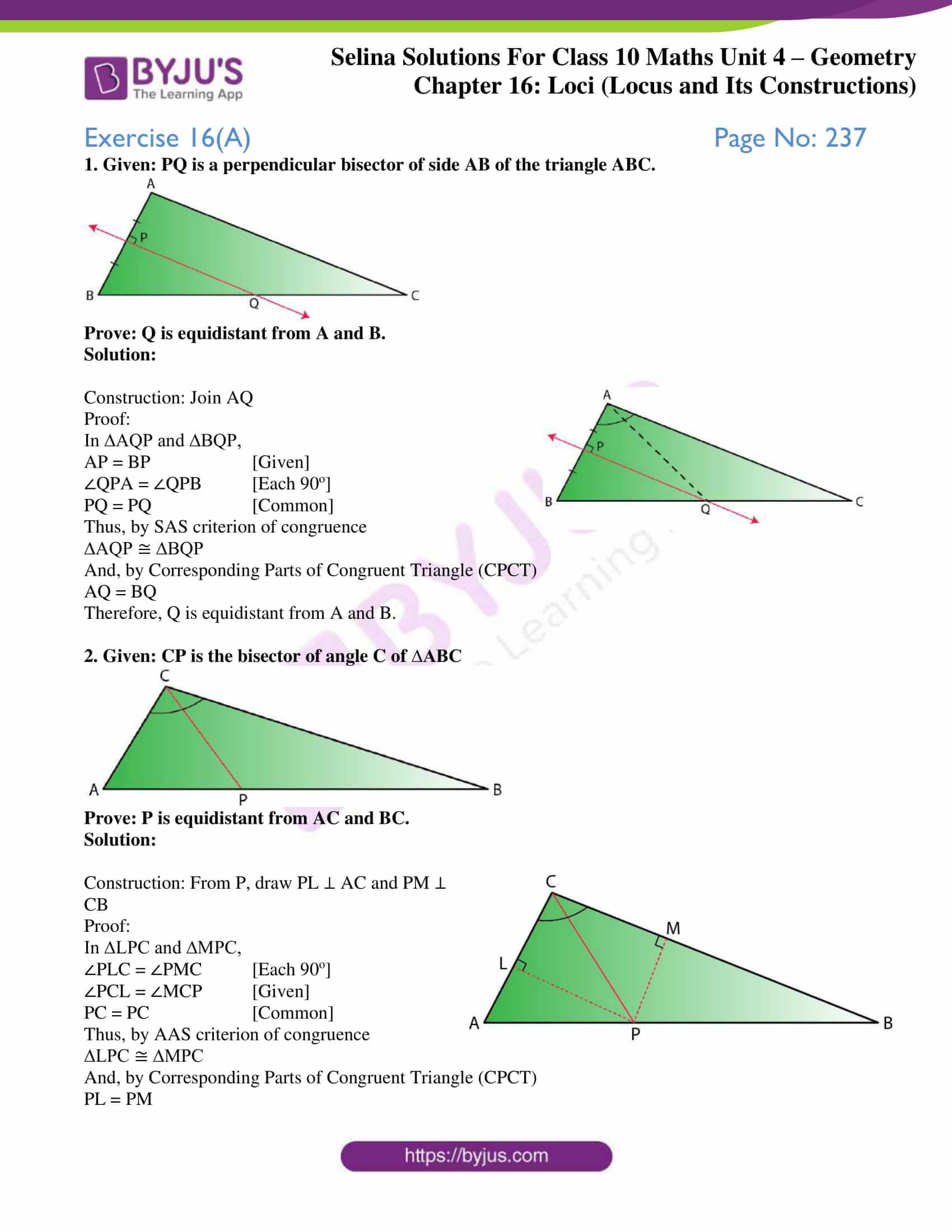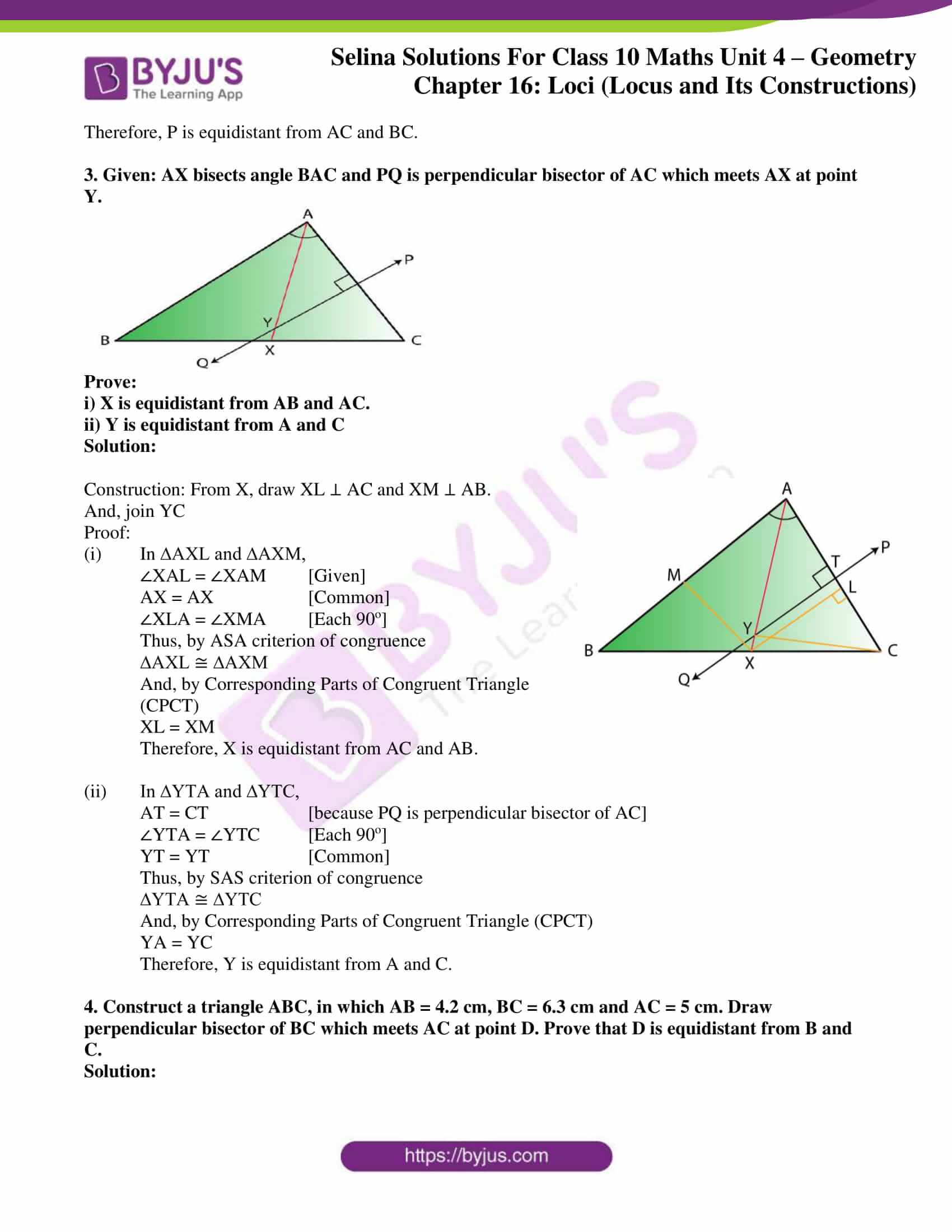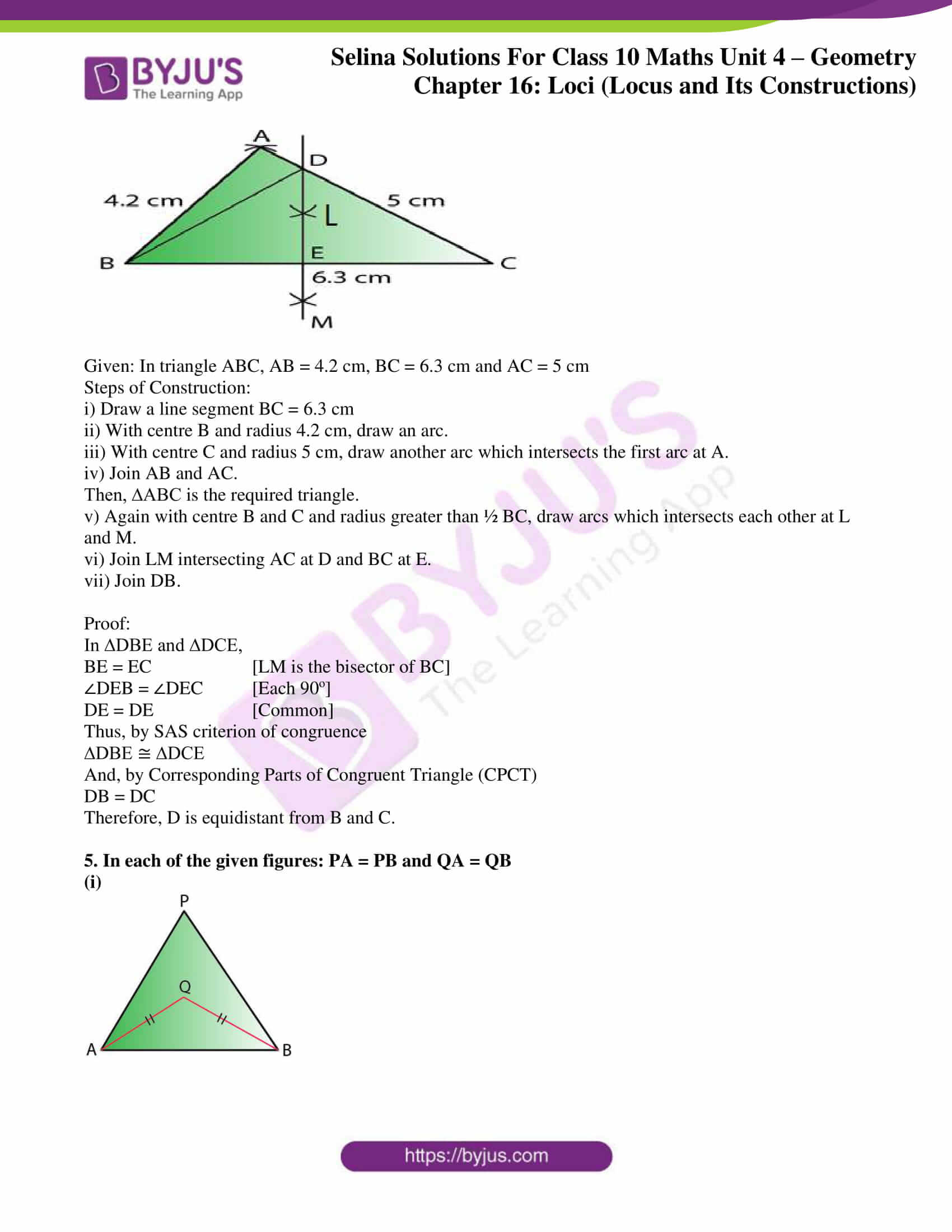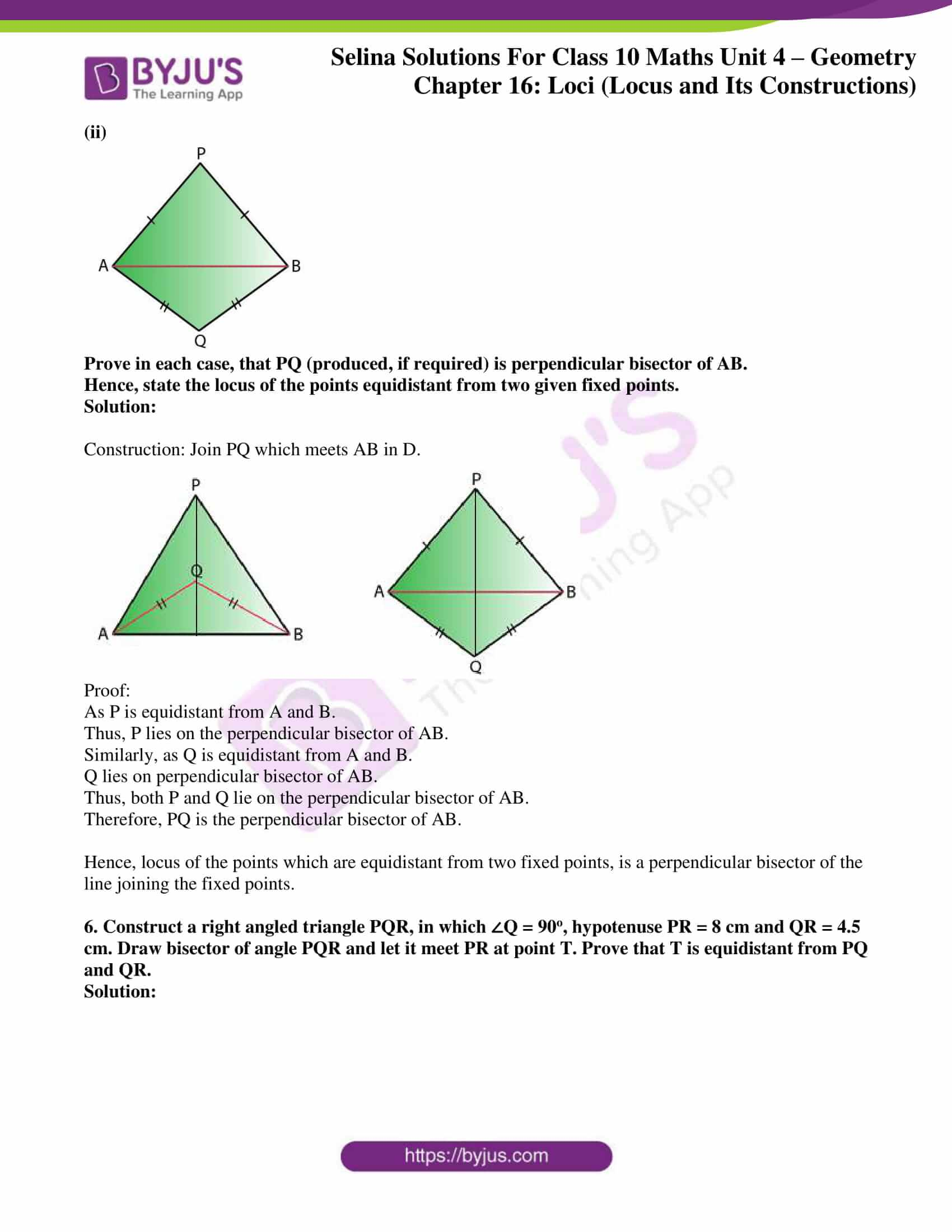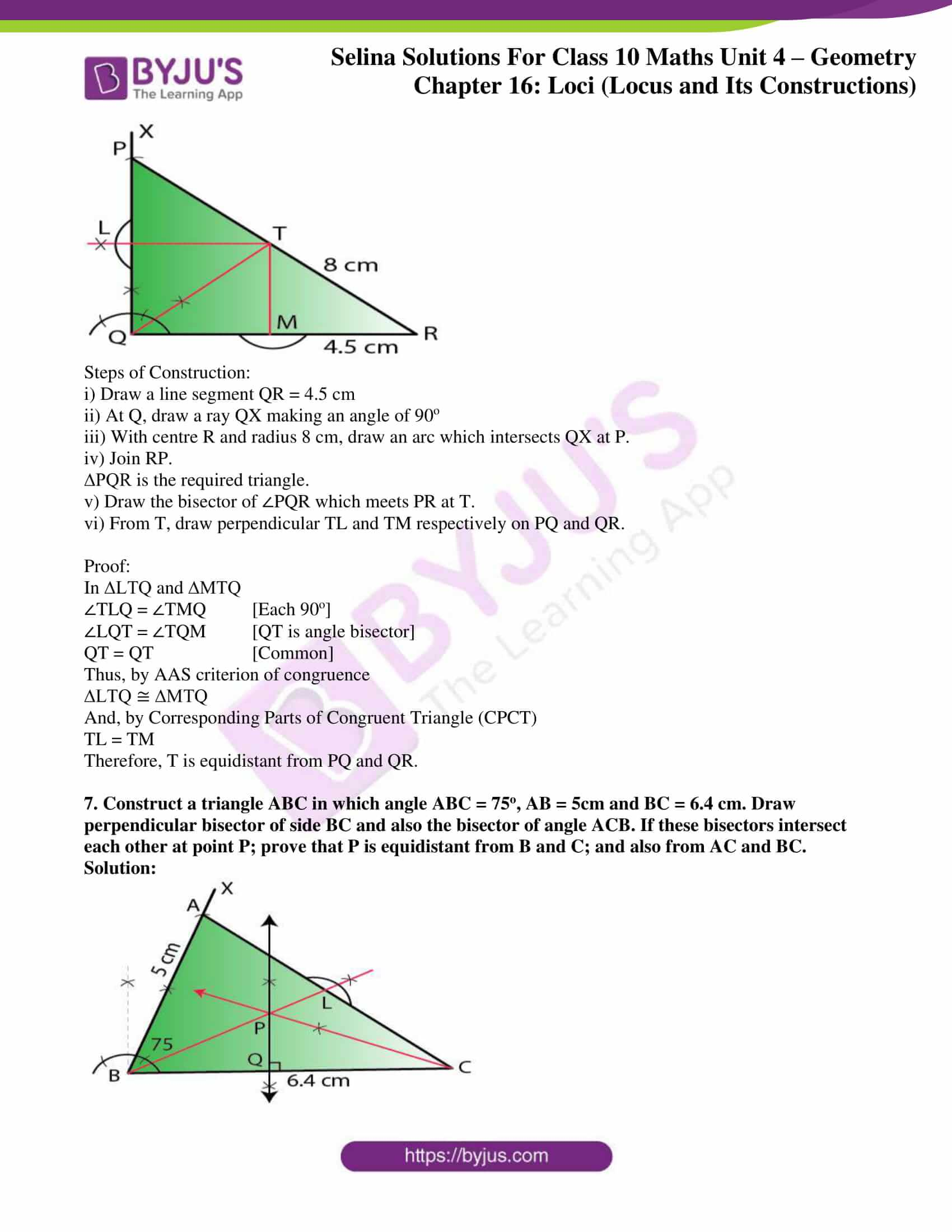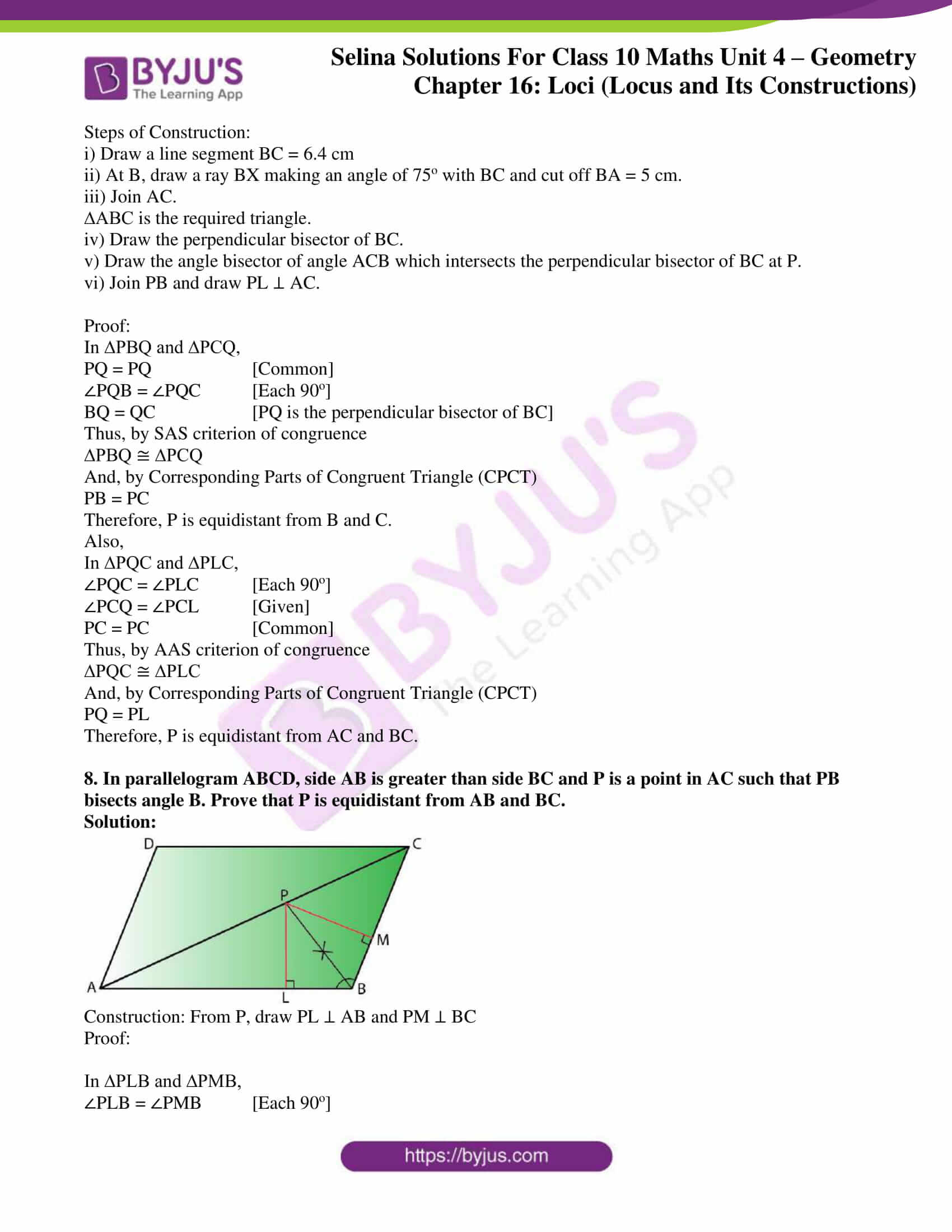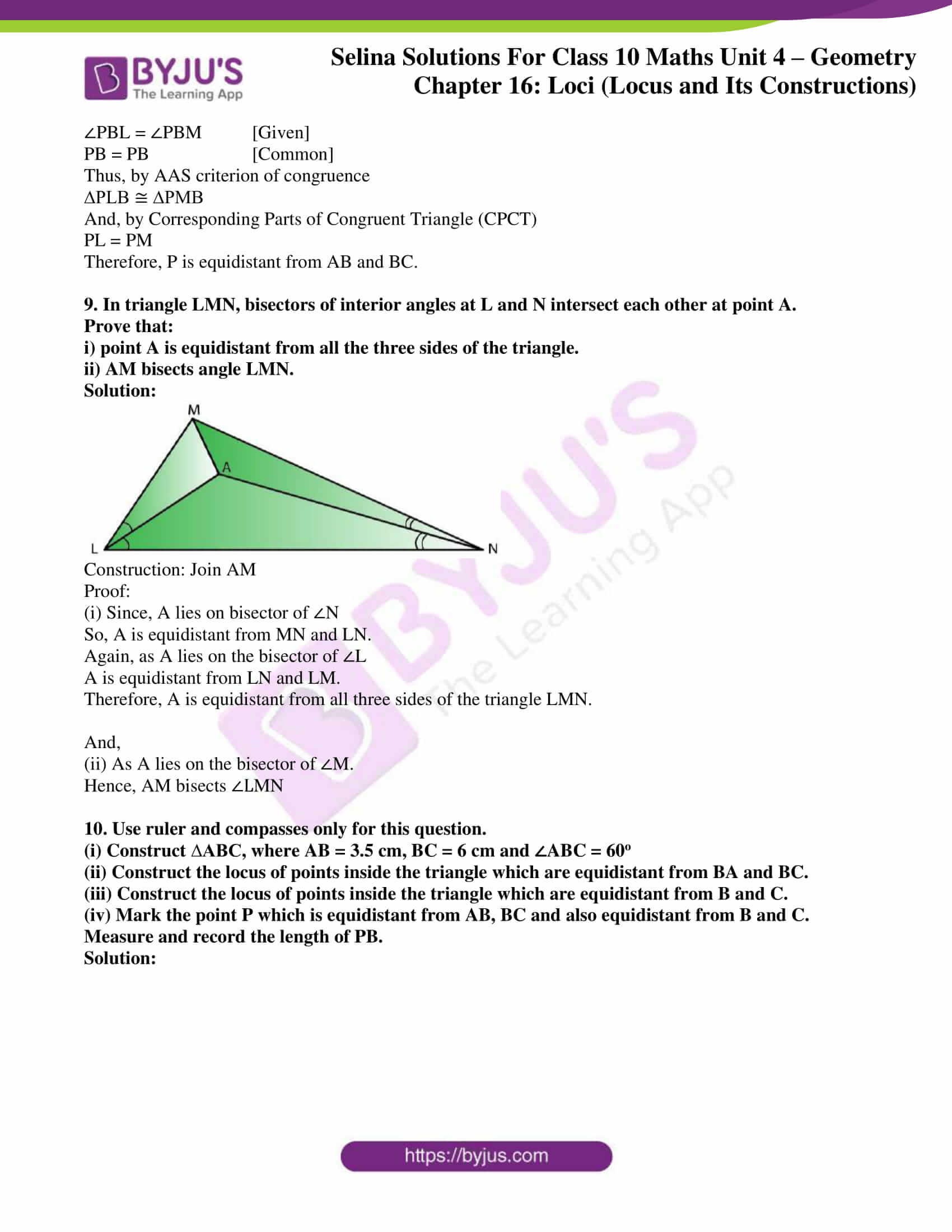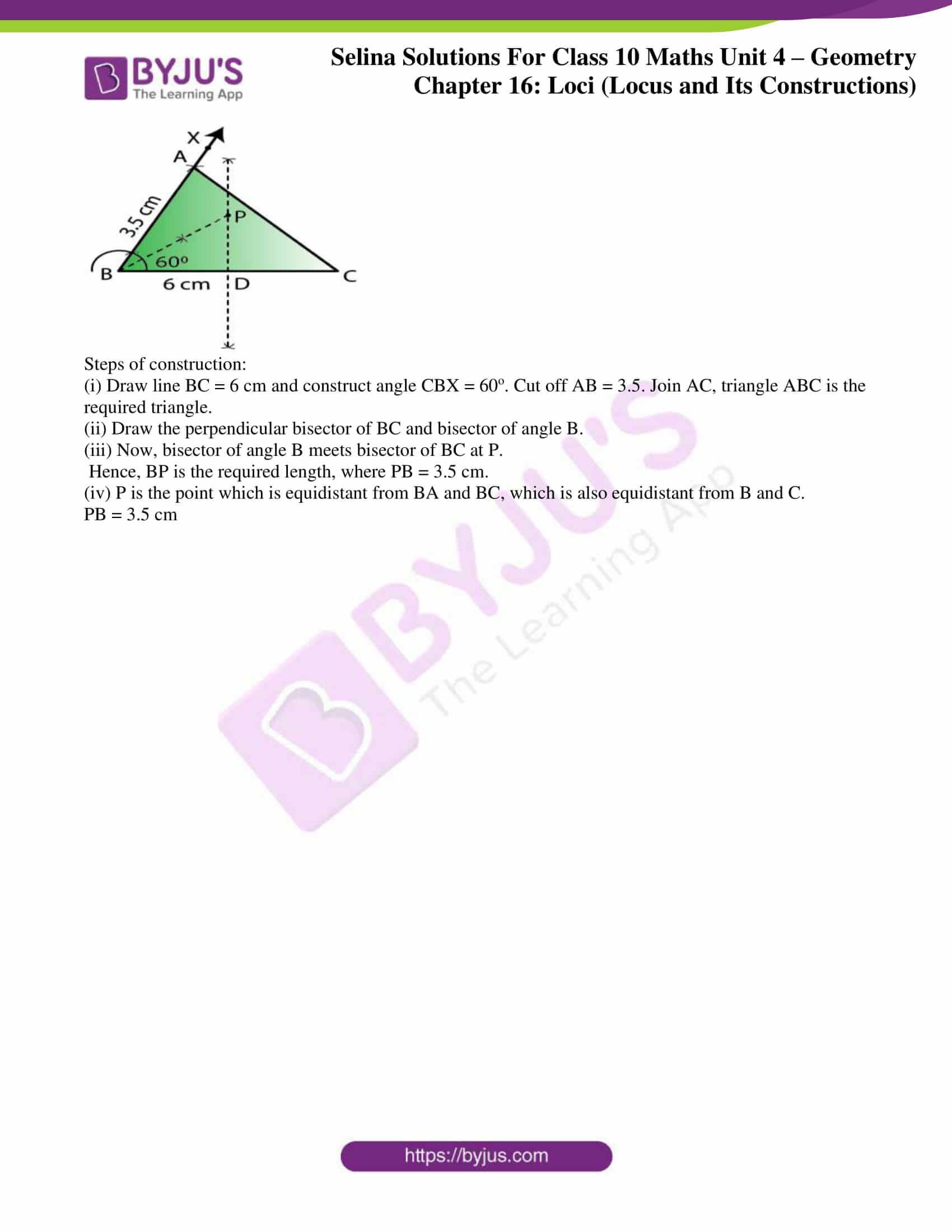### Access Selina Solutions Concise Maths Class 10 Chapter 16 Loci (Locus and its Constructions) Exercise 16(A)

#### Exercise 16(A) Page No: 237

1. Given: PQ is a perpendicular bisector of side AB of the triangle ABC.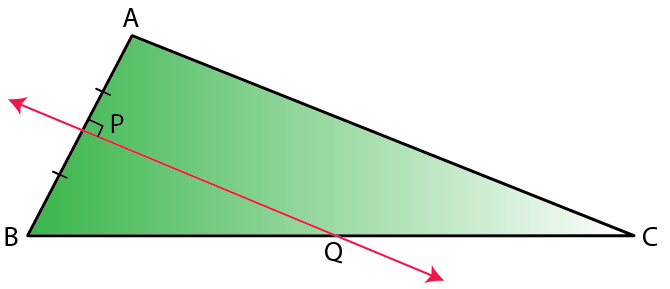Prove: Q is equidistant from A and B.

Solution: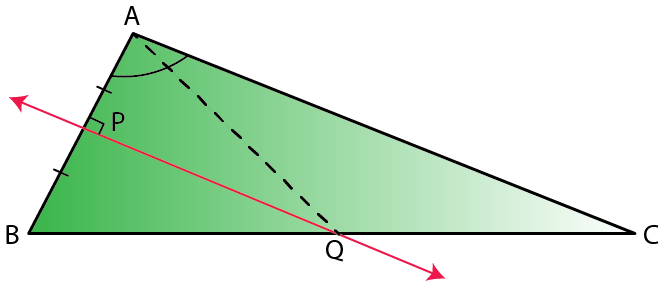Construction: Join AQ

Proof:

In ∆AQP and ∆BQP,

AP = BP [Given]

∠QPA = ∠QPB [Each 90o]

PQ = PQ [Common]

Thus, by SAS criterion of congruence

∆AQP ≅ ∆BQP

And, by Corresponding Parts of Congruent Triangle (CPCT)

AQ = BQ

Therefore, Q is equidistant from A and B.

2. Given: CP is the bisector of angle C of ∆ABC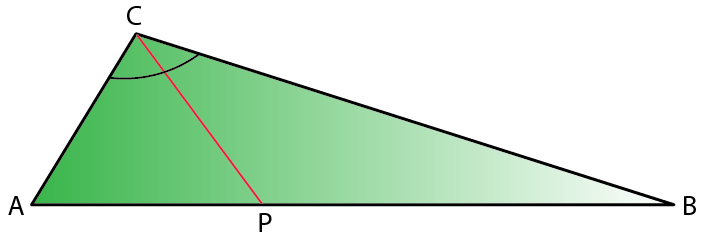Prove: P is equidistant from AC and BC.

Solution: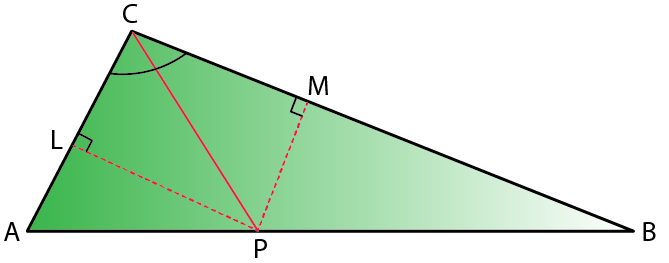Construction: From P, draw PL ⊥ AC and PM ⊥ CB

Proof:

In ∆LPC and ∆MPC,

∠PLC = ∠PMC [Each 90o]

∠PCL = ∠MCP [Given]

PC = PC [Common]

Thus, by AAS criterion of congruence

∆LPC ≅ ∆MPC

And, by Corresponding Parts of Congruent Triangle (CPCT)

PL = PM

Therefore, P is equidistant from AC and BC.

3. Given: AX bisects angle BAC and PQ is perpendicular bisector of AC which meets AX at point Y.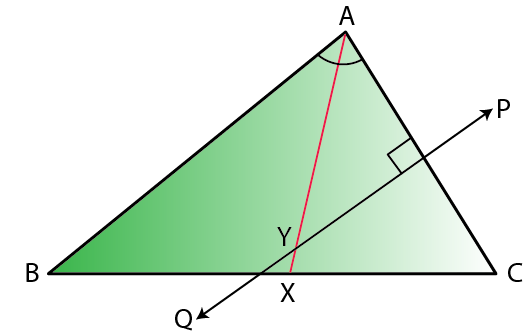Prove:

i) X is equidistant from AB and AC.

ii) Y is equidistant from A and C

Solution: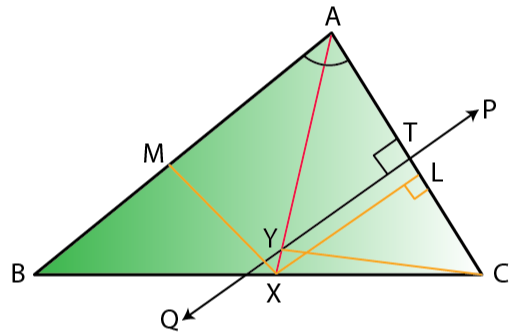Construction: From X, draw XL ⊥ AC and XM ⊥ AB.

And, join YC

Proof:

(i) In ∆AXL and ∆AXM,

∠XAL = ∠XAM [Given]

AX = AX [Common]

∠XLA = ∠XMA [Each 90o]

Thus, by ASA criterion of congruence

∆AXL ≅ ∆AXM

And, by Corresponding Parts of Congruent Triangle (CPCT)

XL = XM

Therefore, X is equidistant from AC and AB.

(ii) In ∆YTA and ∆YTC,

AT = CT [because PQ is perpendicular bisector of AC]

∠YTA = ∠YTC [Each 90o]

YT = YT [Common]

Thus, by SAS criterion of congruence

∆YTA ≅ ∆YTC

And, by Corresponding Parts of Congruent Triangle (CPCT)

YA = YC

Therefore, Y is equidistant from A and C.

4. Construct a triangle ABC, in which AB = 4.2 cm, BC = 6.3 cm and AC = 5 cm. Draw perpendicular bisector of BC which meets AC at point D. Prove that D is equidistant from B and C.

Solution: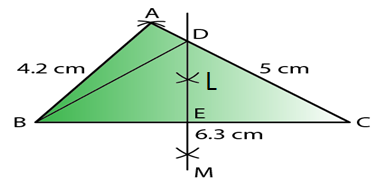Given: In triangle ABC, AB = 4.2 cm, BC = 6.3 cm and AC = 5 cm

Steps of Construction:

i) Draw a line segment BC = 6.3 cm

ii) With centre B and radius 4.2 cm, draw an arc.

iii) With centre C and radius 5 cm, draw another arc which intersects the first arc at A.

iv) Join AB and AC.

Then, ∆ABC is the required triangle.

v) Again with centre B and C and radius greater than ½ BC, draw arcs which intersects each other at L and M.

vi) Join LM intersecting AC at D and BC at E.

vii) Join DB.

Proof:

In ∆DBE and ∆DCE,

BE = EC [LM is the bisector of BC]

∠DEB = ∠DEC [Each 90o]

DE = DE [Common]

Thus, by SAS criterion of congruence

∆DBE ≅ ∆DCE

And, by Corresponding Parts of Congruent Triangle (CPCT)

DB = DC

Therefore, D is equidistant from B and C.

5. In each of the given figures: PA = PB and QA = QB

(i)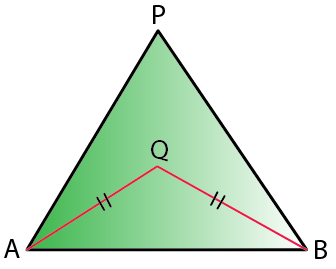(ii)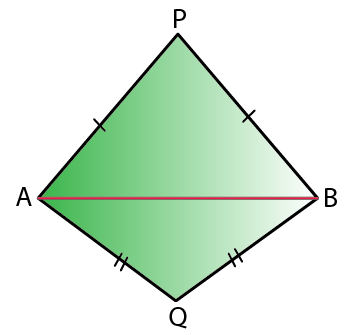Prove in each case, that PQ (produced, if required) is perpendicular bisector of AB.

Hence, state the locus of the points equidistant from two given fixed points.

Solution:

Construction: Join PQ which meets AB in D.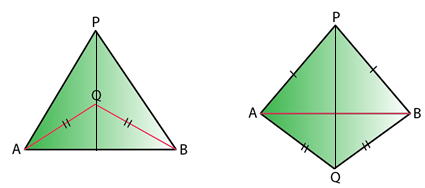Proof:

As P is equidistant from A and B.

Thus, P lies on the perpendicular bisector of AB.

Similarly, as Q is equidistant from A and B.

Q lies on perpendicular bisector of AB.

Thus, both P and Q lie on the perpendicular bisector of AB.

Therefore, PQ is the perpendicular bisector of AB.

Hence, locus of the points which are equidistant from two fixed points, is a perpendicular bisector of the line joining the fixed points.

6. Construct a right angled triangle PQR, in which ∠Q = 90o, hypotenuse PR = 8 cm and QR = 4.5 cm. Draw bisector of angle PQR and let it meet PR at point T. Prove that T is equidistant from PQ and QR.

Solution: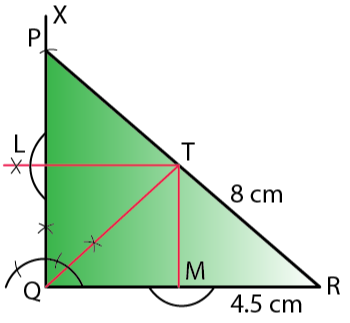Steps of Construction:

i) Draw a line segment QR = 4.5 cm

ii) At Q, draw a ray QX making an angle of 90o

iii) With centre R and radius 8 cm, draw an arc which intersects QX at P.

iv) Join RP.

∆PQR is the required triangle.

v) Draw the bisector of ∠PQR which meets PR at T.

vi) From T, draw perpendicular TL and TM respectively on PQ and QR.

Proof:

In ∆LTQ and ∆MTQ

∠TLQ = ∠TMQ [Each 90o]

∠LQT = ∠TQM [QT is angle bisector]

QT = QT [Common]

Thus, by AAS criterion of congruence

∆LTQ ≅ ∆MTQ

And, by Corresponding Parts of Congruent Triangle (CPCT)

TL = TM

Therefore, T is equidistant from PQ and QR.

7. Construct a triangle ABC in which angle ABC = 75o, AB = 5cm and BC = 6.4 cm. Draw perpendicular bisector of side BC and also the bisector of angle ACB. If these bisectors intersect each other at point P; prove that P is equidistant from B and C; and also from AC and BC.

Solution: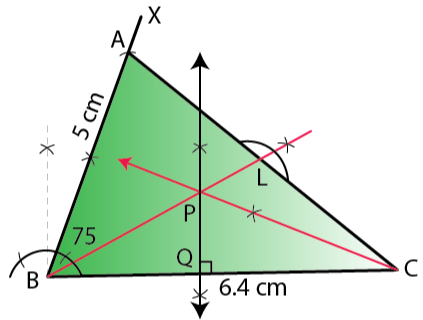Steps of Construction:

i) Draw a line segment BC = 6.4 cm

ii) At B, draw a ray BX making an angle of 75o with BC and cut off BA = 5 cm.

iii) Join AC.

∆ABC is the required triangle.

iv) Draw the perpendicular bisector of BC.

v) Draw the angle bisector of angle ACB which intersects the perpendicular bisector of BC at P.

vi) Join PB and draw PL ⊥ AC.

Proof:

In ∆PBQ and ∆PCQ,

PQ = PQ [Common]

∠PQB = ∠PQC [Each 90o]

BQ = QC [PQ is the perpendicular bisector of BC]

Thus, by SAS criterion of congruence

∆PBQ ≅ ∆PCQ

And, by Corresponding Parts of Congruent Triangle (CPCT)

PB = PC

Therefore, P is equidistant from B and C.

Also,

In ∆PQC and ∆PLC,

∠PQC = ∠PLC [Each 90o]

∠PCQ = ∠PCL [Given]

PC = PC [Common]

Thus, by AAS criterion of congruence

∆PQC ≅ ∆PLC

And, by Corresponding Parts of Congruent Triangle (CPCT)

PQ = PL

Therefore, P is equidistant from AC and BC.

8. In parallelogram ABCD, side AB is greater than side BC and P is a point in AC such that PB bisects angle B. Prove that P is equidistant from AB and BC.

Solution: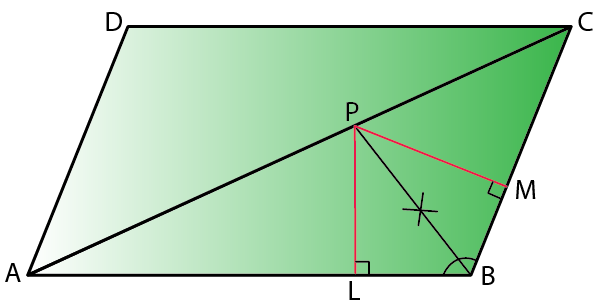Construction: From P, draw PL ⊥ AB and PM ⊥ BC

Proof:

In ∆PLB and ∆PMB,

∠PLB = ∠PMB [Each 90o]

∠PBL = ∠PBM [Given]

PB = PB [Common]

Thus, by AAS criterion of congruence

∆PLB ≅ ∆PMB

And, by Corresponding Parts of Congruent Triangle (CPCT)

PL = PM

Therefore, P is equidistant from AB and BC.

9. In triangle LMN, bisectors of interior angles at L and N intersect each other at point A.

Prove that:

i) point A is equidistant from all the three sides of the triangle.

ii) AM bisects angle LMN.

Solution: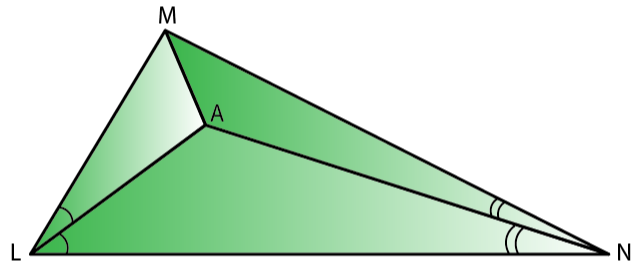Construction: Join AM

Proof:

(i) Since, A lies on bisector of ∠N

So, A is equidistant from MN and LN.

Again, as A lies on the bisector of ∠L

A is equidistant from LN and LM.

Therefore, A is equidistant from all three sides of the triangle LMN.

And,

(ii) As A lies on the bisector of ∠M.

Hence, AM bisects ∠LMN

10. Use ruler and compasses only for this question.

(i) Construct ∆ABC, where AB = 3.5 cm, BC = 6 cm and ∠ABC = 60o

(ii) Construct the locus of points inside the triangle which are equidistant from BA and BC.

(iii) Construct the locus of points inside the triangle which are equidistant from B and C.

(iv) Mark the point P which is equidistant from AB, BC and also equidistant from B and C. Measure and record the length of PB.

Solution: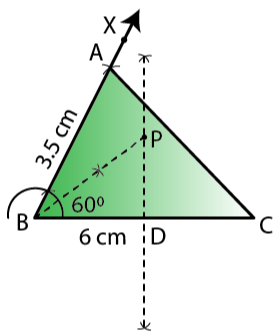Steps of construction:

(i) Draw line BC = 6 cm and construct angle CBX = 60o. Cut off AB = 3.5. Join AC, triangle ABC is the required triangle.

(ii) Draw the perpendicular bisector of BC and bisector of angle B.

(iii) Now, bisector of angle B meets bisector of BC at P.

Hence, BP is the required length, where PB = 3.5 cm.

(iv) P is the point which is equidistant from BA and BC, which is also equidistant from B and C.

PB = 3.5 cm

### Access another exercise of Selina Solutions Concise Maths Class 10 Chapter 16 Loci (Locus and its Constructions)

Exercise 16(B) Solutions